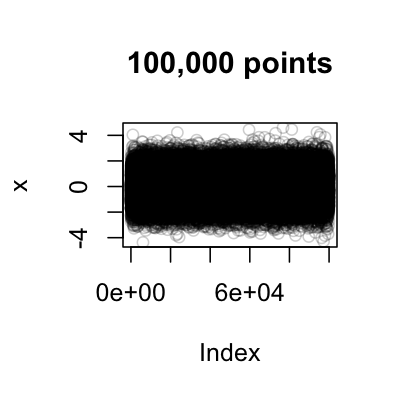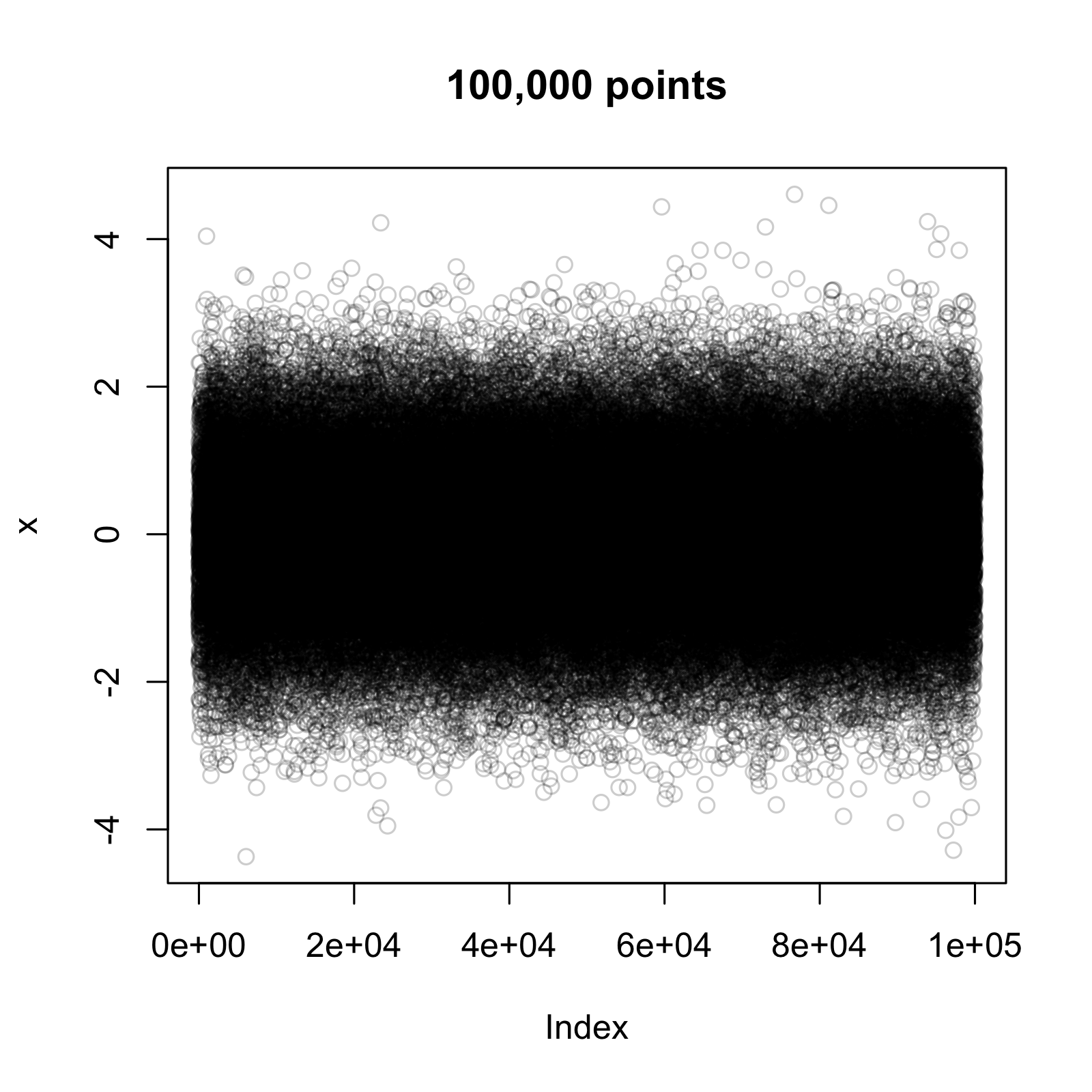High resolution graphics with R

For most purposes PDF or other vector graphic formats such as windows metafile and SVG work just fine. However, if I plot lots of points, say 100k, then those files can get quite large and bitmap formats like PNG can be the better option. I just have to be mindful of the resolution.

As an example I create the following plot:

x <- rnorm(100000)plot(x, main=“100,000 points”, col=adjustcolor(“black”, alpha=0.2))
Saving the plot as a PDF creates a 5.2 MB big file on my computer, while the PNG output is only 62 KB instead. Of course, the PNG doesn’t look as crisp as the PDF file.
png(“100kPoints72dpi.png”, units = “px”, width=400, height=400)plot(x, main=“100,000 points”, col=adjustcolor(“black”, alpha=0.2))dev.off()Hence, I increase the resolution to 150 dots per pixel.
png(“100kHighRes150dpi.png”, units=“px”, width=400, height=400, res=150)plot(x, main=“100,000 points”, col=adjustcolor(“black”, alpha=0.2))dev.off()This looks a bit odd. The file size is only 29 KB but the annotations look too big. Well, the file has only 400 x 400 pixels and the size of a pixel is fixed. Thus, I have to provide more pixels, or in other words increase the plot size. Doubling the width and height as I double the resolution makes sense.
png(“100kHighRes150dpi2.png”, units=“px”, width=800, height=800, res=150)plot(x, main=“100,000 points”, col=adjustcolor(“black”, alpha=0.2))dev.off()Next I increase the resolution further to 300 dpi and the graphic size to 1600 x 1600 pixels. The file is still very crisp. Of course the file size increased. Now it is 654 KB in size, yet sill only about 18 of the PDF and I can embed it in LaTeX as well.
png(“100kHighRes300dpi.png”, units=“px”, width=1600, height=1600, res=300)plot(x, main=“100,000 points”, col=adjustcolor(“black”, alpha=0.2))dev.off()